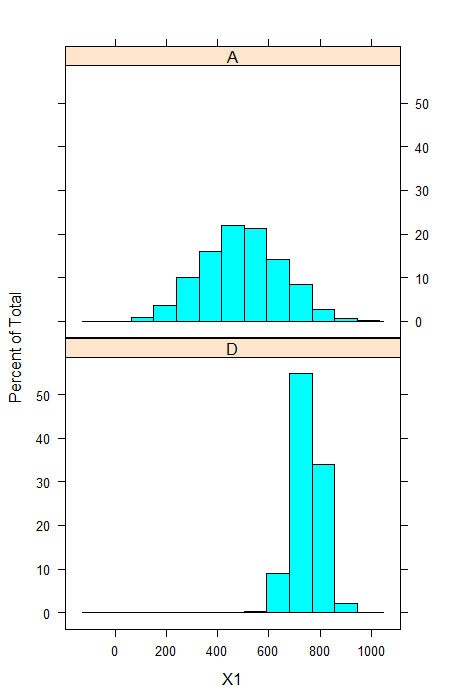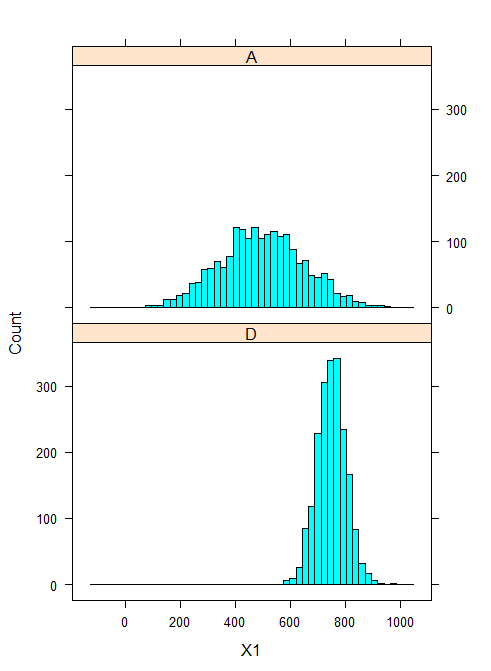# R cran Stacked Histograms

Stacking histograms using the lattice library:

```library(lattice)
histogram(~X1|V1, data = df0, layout=c(1,2))```

Which produces something like this (you’ll need to adjust the layout parameter if there are more factor levels):Some more options:

```histogram(~X1|V1
, data = df0
, layout=c(1,2) ##stack them
, type="count" ##also available are percentage and density
, nint=50 ##number of bins
)```

Which produces this: